# SilverZone - iRAO PDF Sample Papers for Class 9

Class 9 sample paper & practice questions for International Reasoning & Aptitude Olympiad (iRAO) level 1 are given below. Syllabus for level 1 is also mentioned for these exams. You can refer these sample paper & quiz for preparing for the exam.#### Resources:

##### Sample Questions from Olympiad Success:
 Q.1 Q.2 Q.3 Q.4 Q.5 Q.6 Q.7 Q.8 Q.9 Q.10
 Q.1 Use figure to solve questions In a rectangle, the diagonals intersect at a point. If angle A=60°, then E= ______.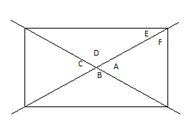a) 30° b) 60° c) 90° d) 0°
 Q.2 n is a positive integer. Let's say that {n} denotes the sum of all the positive divisors of n except for n itself. For example, {4} = 1 + 2 = 3 and <8> = 1+2+4 = 7. What is {{{496}}}? a) 1 b) 496 c) 495 d) 497
 Q.3 Which number will replace the question mark?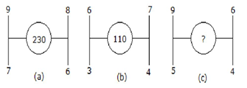a) 154 b) 156 c) 160 d) 158
 Q.4 There is a line AB; C is the midpoint of AB and D is the midpoint of AC. Now CD = CB/2. Then CD + AD =? a) AB b) BD c) CD d) CB
 Q.5 The number of boys in a class is three times the number of girls. Which one of the following numbers cannot represent the total number of children in the class? a) 54 b) 56 c) 64 d) 72
 Q.6 The hypotenuse of a right angle triangle which is common to both the triangles in a square is _____ to square. a) Parallel b) Perpendicular c) Diagonal d) Transversal
 Q.7 Choose the alternative which closely resembles the mirror image of the given combination.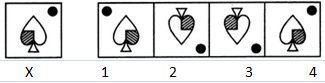a) 1 b) 2 c) 3 d) 4
 Q.8 A (2, 5), B (-1, 2) and C (5, 8) are the vertices of a triangle. P and Q are points on AB and Ac respectively such that AP: PB=AQ: QC=1:2. Coordinates of P and Q are a) (1, 4) and (6, 3) b) (1, 4) and (3, 6) c) (4, 1) and (3, 6) d) (4, 1) and (6, 3)
 Q.9 If a cube is coloured red on one face, green on the opposite face, yellow on another face and blue on a face adjacent to the yellow face. The other two faces left uncoloured. It is cut into 125 smaller cubes of equal size. How many cubes are uncolored on all faces? a) 1 b) 27 c) 8 d) 24
 Q.10 Which figure satisfy the placement of dots?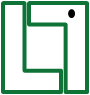a)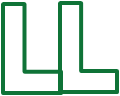b)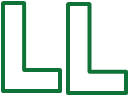c)d)Sample PDF of SilverZone - International Reasoning & Aptitude Olympiad (iRAO) PDF Sample Papers for Class 9:

 Q.1 )a Q.2 )b Q.3 )d Q.4 )d Q.5 )a Q.6 )c Q.7 )a Q.8 )c Q.9 )b Q.10 )b

Q.1 : a | Q.2 : b | Q.3 : d | Q.4 : d | Q.5 : a | Q.6 : c | Q.7 : a | Q.8 : c | Q.9 : b | Q.10 : b